## Heap Sort Back

### Overview

• 堆排序: 利用大頂堆或小頂堆的特性, 每趟取出樹根結點並調整堆, 直到所有數被取出.
• 大頂堆: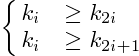• 小頂堆: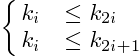• 时间复杂度: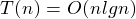(最好,平均,最壞情況)
• 空間複雜度: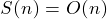• 稳定性: 不稳定
• 适用情况: 實時應用, 快速取出最大或最小值
• BUILD_MAX_HEAP: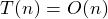• MAX_HEAPIFY: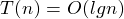### Example

##### 1. BUILD_MAX_HEAP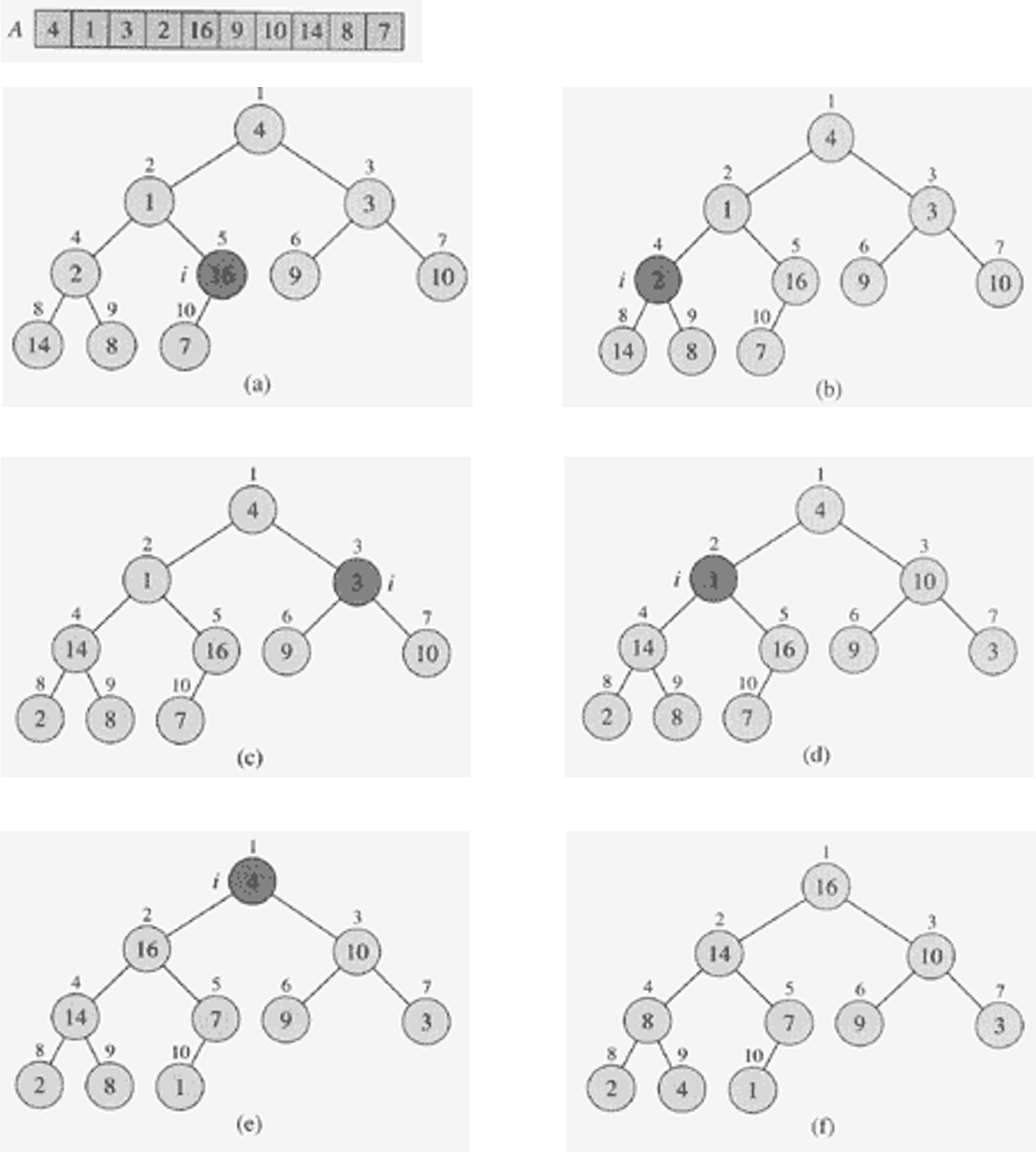##### 2. MAX_HEAPIFY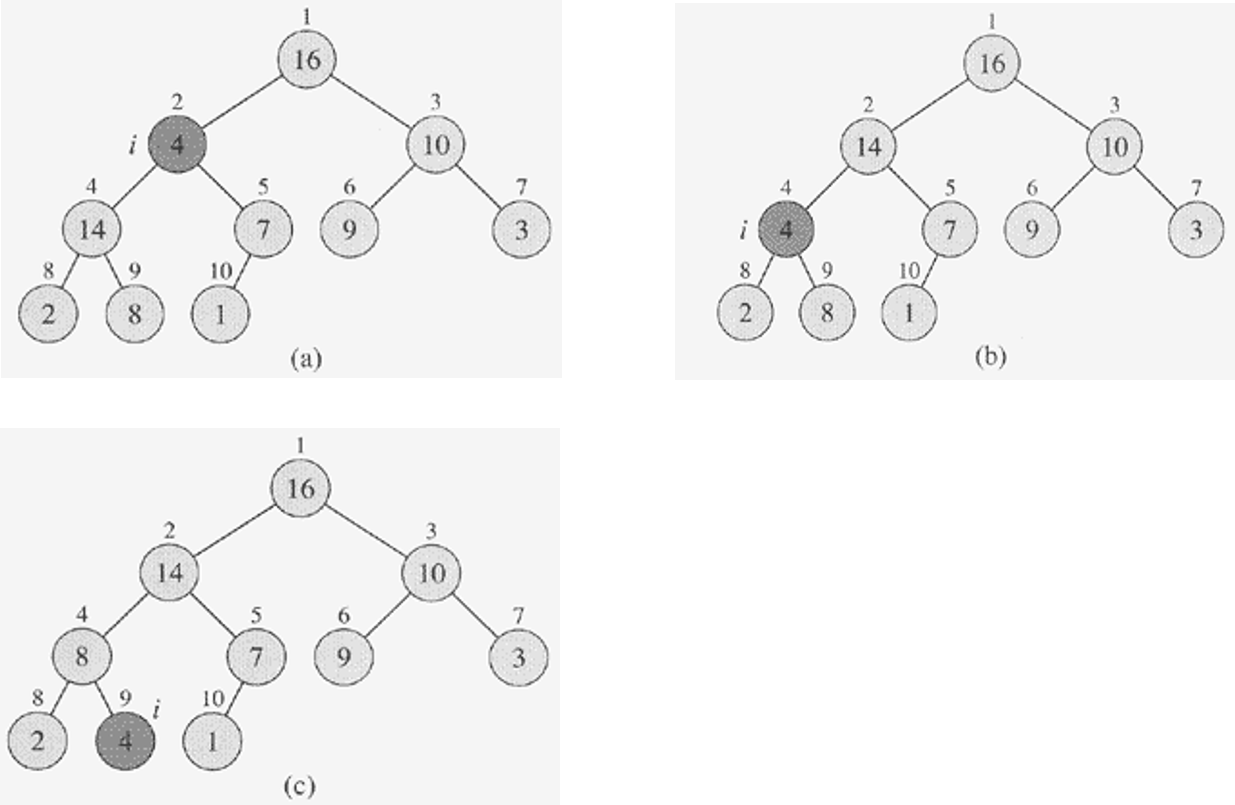##### 3. HEAP_SORT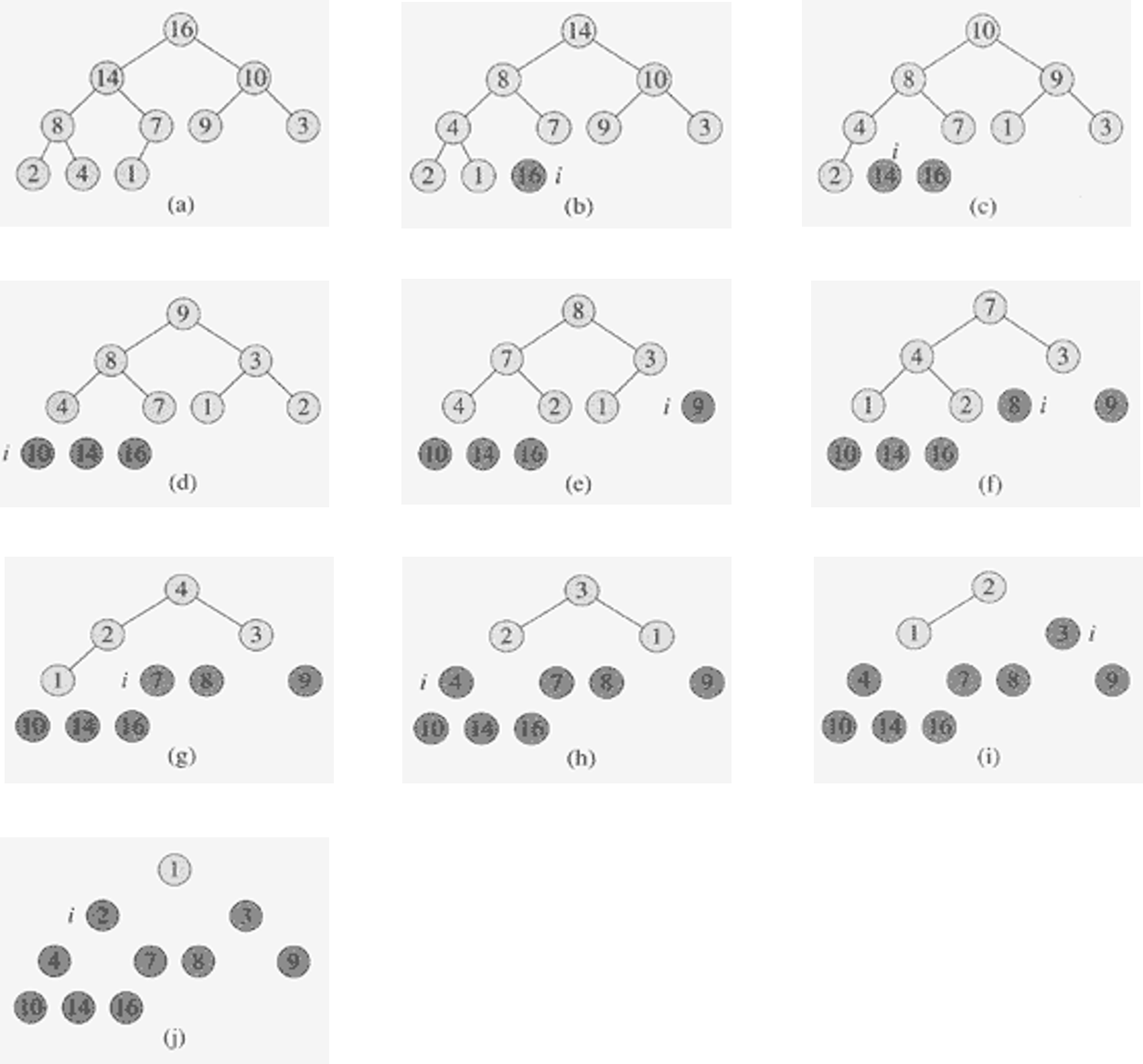### Code

``````void MAX_HEAPIFY(int A[], int heap_size, int i)
{
int largest;
int l = LEFT(i);
int r = RIGHT(i);
if (l < heap_size && A[l] > A[i])
largest = l;
else
largest = i;

if (r < heap_size && A[r] > A[largest])
largest = r;
if (largest != i)
{
exchange(A, i, largest);
MAX_HEAPIFY(A, heap_size, largest);
}
}

void BUILD_MAX_HEAP(int A[], int heap_size)
{
for (int i = heap_size / 2 - 1; i >= 0; i--)
MAX_HEAPIFY(A, heap_size, i);
}

void HEAPSORT(int A[], int* heap_size)
{
BUILD_MAX_HEAP(A,*heap_size);

for (int i = *heap_size - 1; i > 0; i--)
{
exchange(A, 0, i);
*heap_size = *heap_size - 1;
MAX_HEAPIFY(A, *heap_size, 0);
}
}
``````